cancel
Showing results for
Did you mean:

# Code ExamplesHonored Contributor II

## RMS measurement using window function

Attached below is a demo project showing RMS (Root Mean Square) measurement using weighting function technique. In such approach ADC is continuously sampling the signal, and RMS is calculated over finite interval of ~10 AC periods using a weighted window. This simple approach works if signal frequency variations are within pre-defined range, and high output rate is not needed. It can work for non-demanding application such as 50-60 Hz AC monitoring.

The idea is illustrated on Chart 1.

Chart 1. Calculated AC signal, signal squared, weighting window and their product.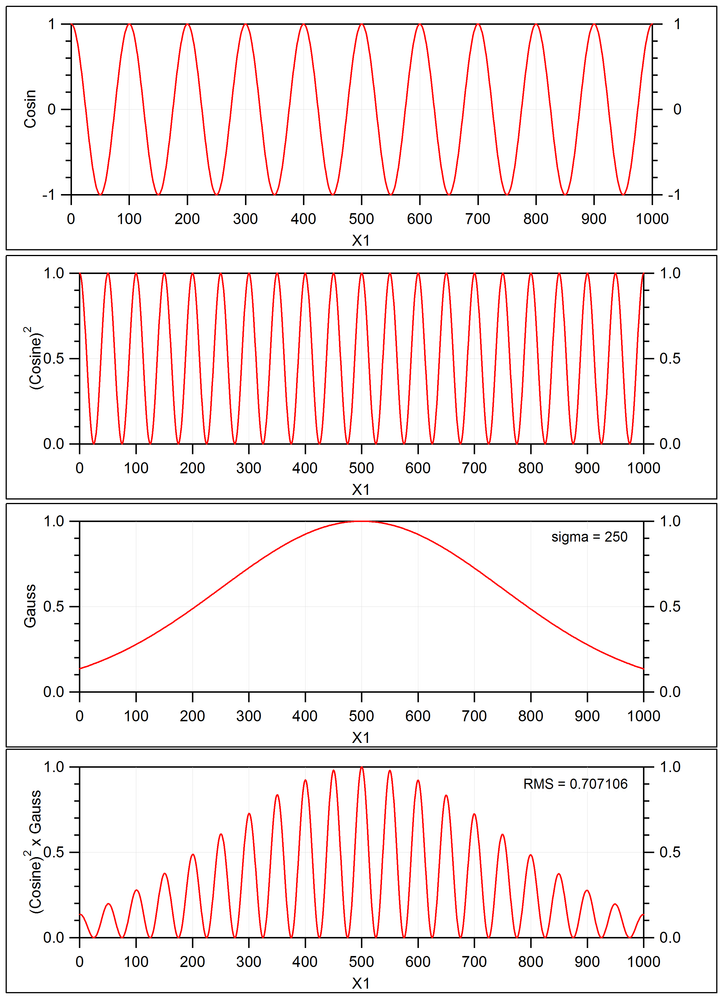AC signal simulated by the Cosine (10 periods), and weighting function by the Gaussian, calculated over 1001 points (1000 intervals).

Cosine = cos( 2 * PI * Freq *  X ); Gauss = exp( -(X^2 / (2 * sigma^2) ), and where Freq = 10 and sigma = 250. The pseudocode is provided here:

Re: bounces happened at comparator output

Despite the weighting function here is being clipped, the calculated RMS = 0.707106 matches theoretical value sqrt(1/2).

The project uses double-buffering technique. The incoming AC signal is continuously sampled using PSoC5 DeltaSigma ADC (16-bit) and transferred to the ring Buffer in RAM by DMA. Once half of Buffer is filled, the interrupt is fired requesting data processing, while DMA continues to populate the Buffer in the background. RMS update frequency is defined by the size of the Buffer and ADC sampling rate. ADC sampling rate determines amount of harmonics captured, thus the accuracy of the measurements. Note that this approach does not require synchronizing the ADC sampling clock and AC signal, which makes it very simple and portable to PSoC4 and PSoC6.

Data below shown for ADC sampling rate of 2 kHz and Buffer size of 2x401 samples. Resulting RMS update rate was approx. 5 Hz. Project was also tested using other parameters: ADC-1, 2, 4, 8 kHz, 1/2 Buffer size - 201, 401 and 801 samples.

Project includes optional arbitrary signal generator, which can be safely removed if external signal generator is available. It requires following custom components:

*   DDS32 v0.0                  : Arbitrary frequency DDS generator. https://community.cypress.com/message/158566#158566

*   WaveGen8 v0.0 (beta) : Arbitrary shape wave generator (included into this project)

Other optional custom component used in the project:

*   PSoC Annotation Library v1.0: https://community.cypress.com/message/204321

*   StopWatch v0.0 (beta)  : Timer for code profiling (included into this project)

Also attached a stripped down version of the project (without signal generator and other external components). This may help if external signal generator is available.

/odissey1

Figure 1. Project schematic.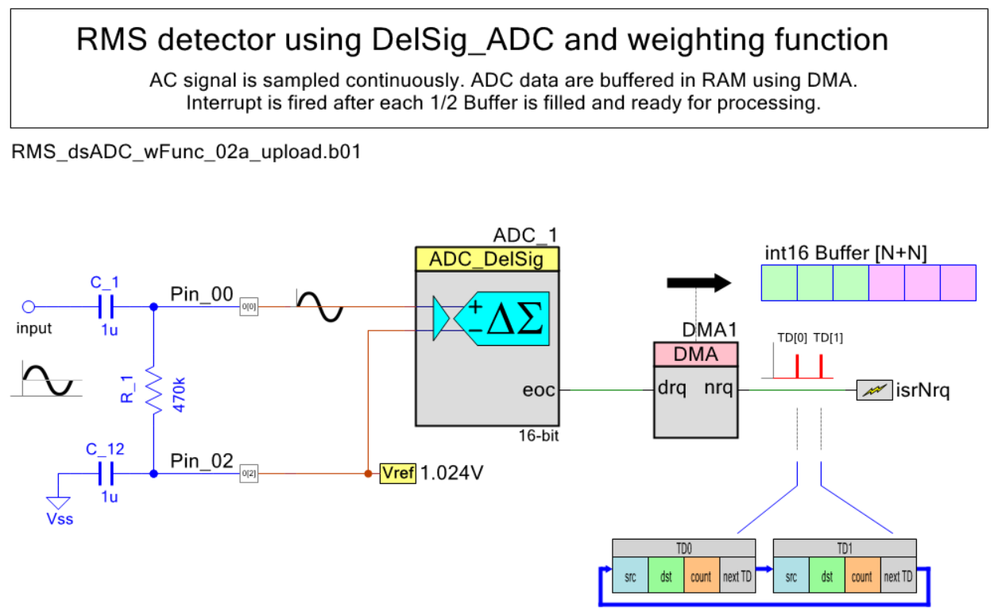Figure 2. Project timing diagram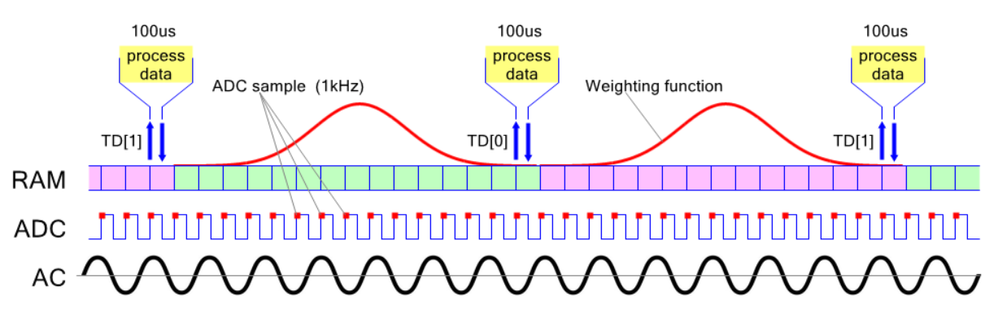Figure 3. Optional arbitrary signal generator.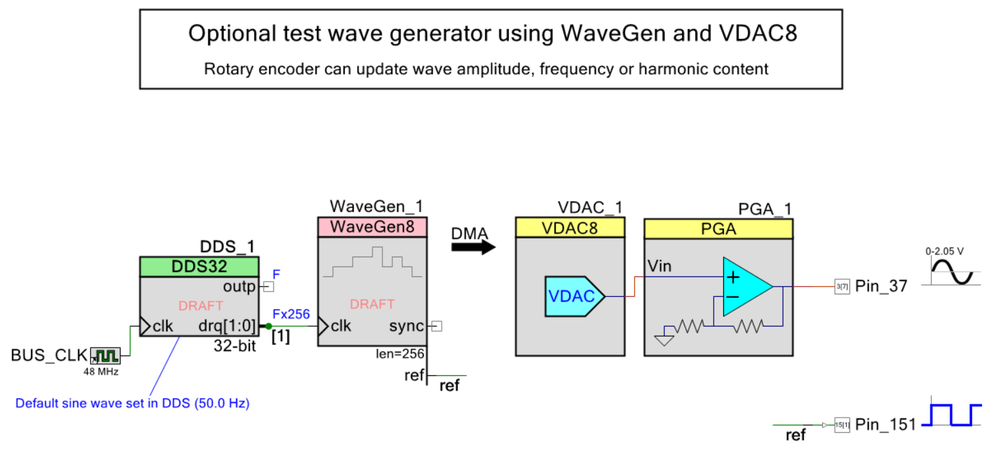Figure 4. KIT-059 Circuit diagram using PSoC Annotation Library v1.0.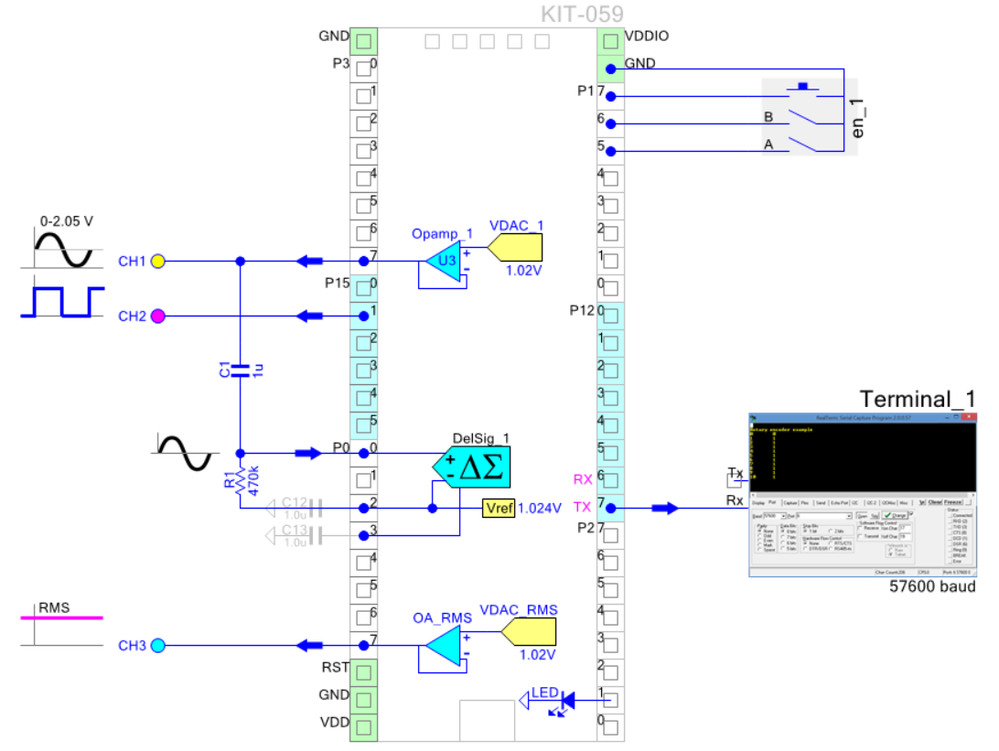Figure 5. Yellow trace - AC signal input; Cyan - signal reference; Fuchsia - RMS voltage output.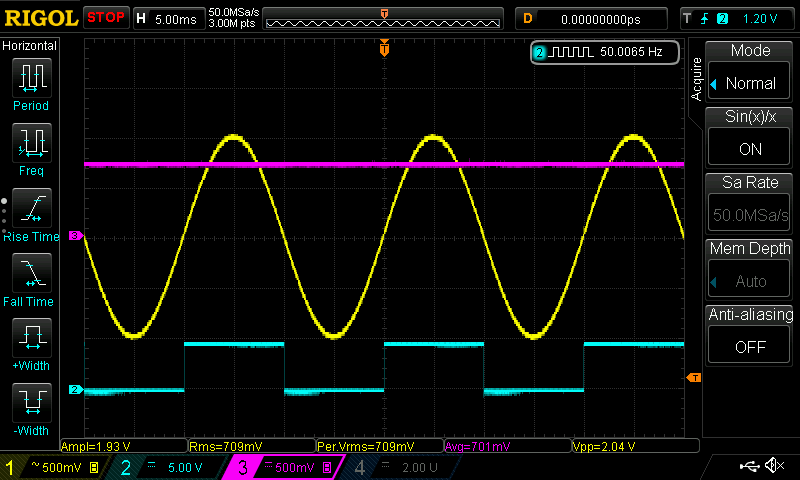Figure 6. UART terminal output.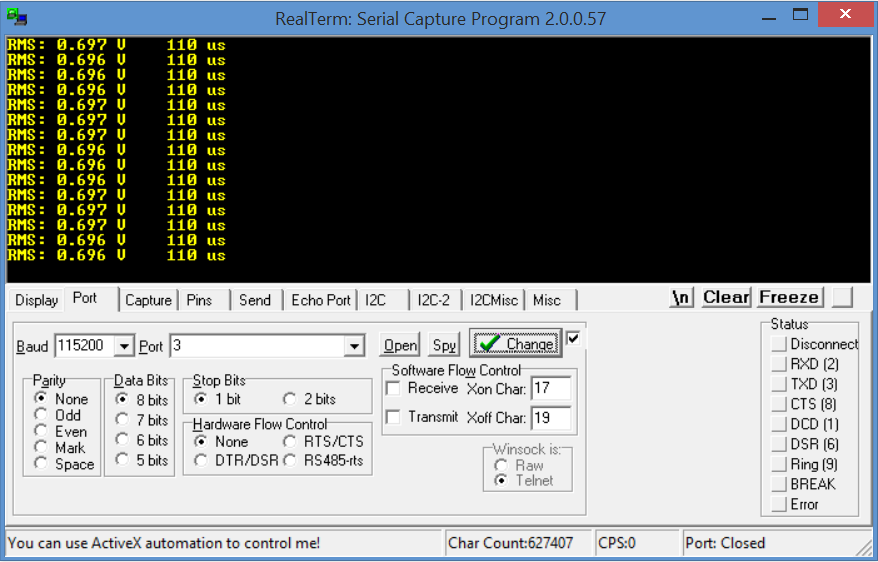17 RepliesHonored Contributor II

## Re: RMS measurement using window function

With some tweaking the project can be improved little further. Attached demo doubles RMS reporting speed (20 Hz) by eliminating sampling gaps and improves standard deviation of the measurement by an order of magnitude. This has been achieved by using quad-buffered DMA (instead of double-buffered), and utilizing the Hanning window (instead of clipped Gaussian): Window = 0.5 - 0.5 Cos( 2 * PI * i / N );

Attached also a stripped down version of the project (no custom components). It requires external signal generator for testing.

Data shown for ADC sampling rate of 4 kHz and Buffer size of 4x200 samples. Resulting RMS update rate was 20 Hz. RMS stability was better then 1 mV. Upper AC frequency was 280 Hz (1% error). Lower frequency threshold is 10 Hz (below that RMS begins to follow DC). Project uses very little resources. Estimated processor load is 0.25%.

/odissey1

Figure 1. Project schematic.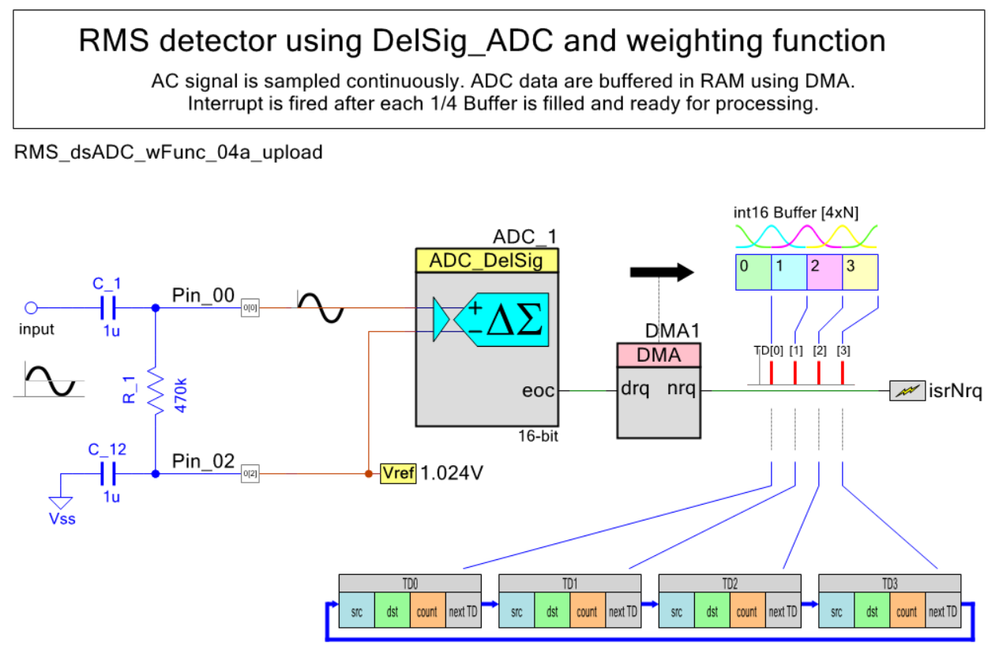Figure 2. Project timing diagram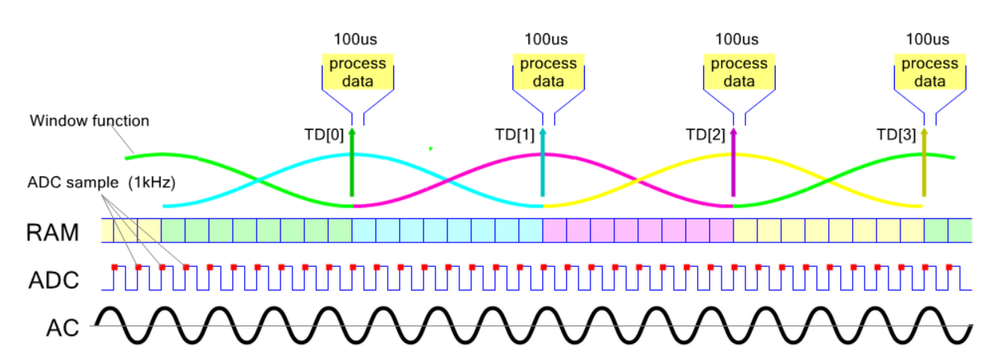Figure 3. Yellow - AC signal input (50Hz); Cyan - signal reference; Fuchsia - RMS output.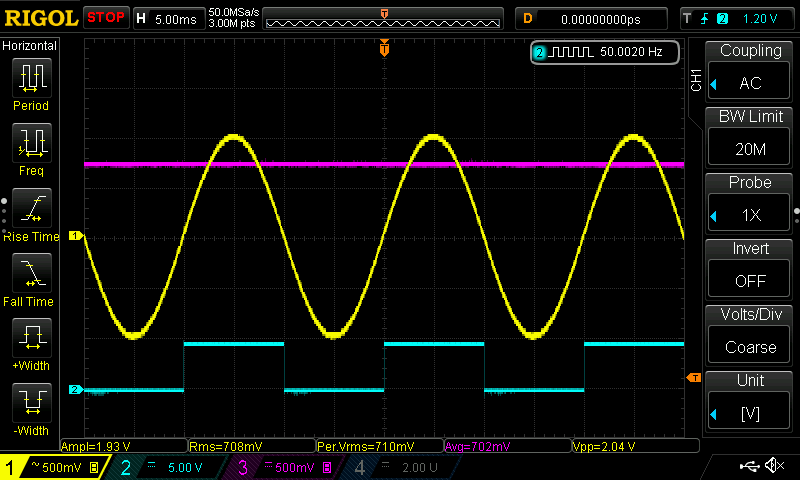Figure 4. Yellow - AC signal input (280Hz); Cyan - signal reference; Fuchsia - RMS output.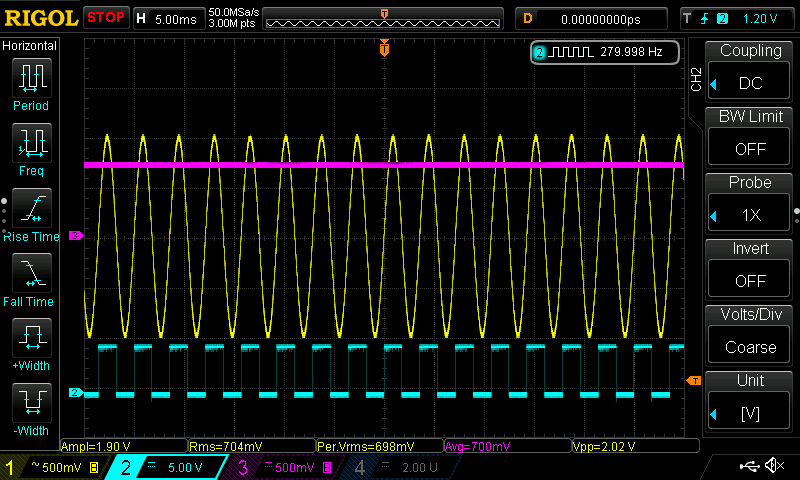Figure 5. Yellow - AC signal input (50Hz); Cyan - signal reference; Fuchsia - RMS output.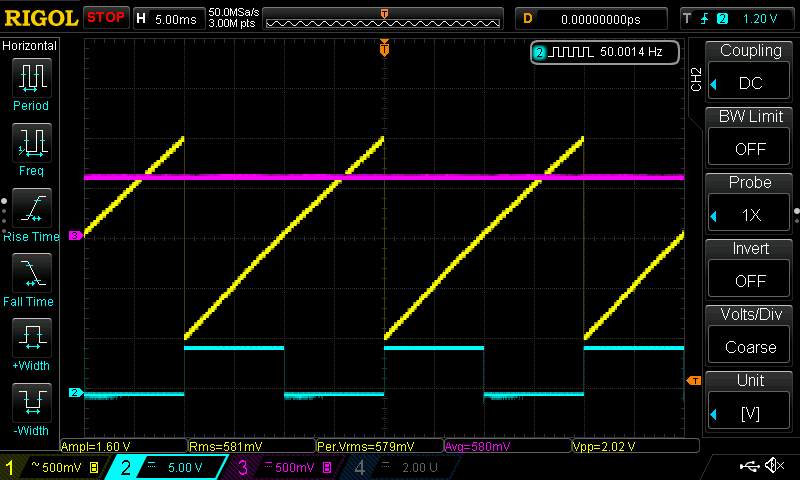Figure 6. Calculated AC signal, signal squared, the Hanning window and their product.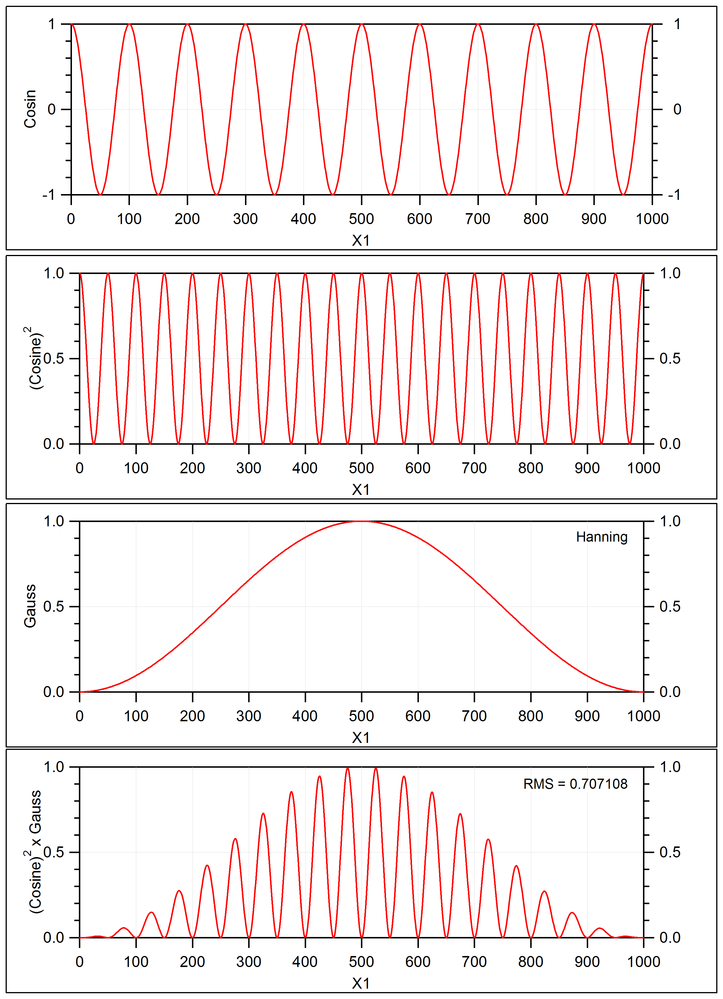New Contributor II

## Re: RMS measurement using window function

Hello,

Is the author of this post still active? I'm wondering if this approach can be implemented with a SAR 12-bit ADC.Honored Contributor II

## Re: RMS measurement using window function

jigr,

I am still alive, but my computer is not, it is in repair stage.

The RMS measurement will work using SAR_ADC as well. The only thing which need to be changed is the DMA source pointer. Let me know if you need any help with that.

/odissey1New Contributor II

## Re: RMS measurement using window function

Thanks for the response. I kind of need some help. Could you explain a bit more on the DMA source pointer? Your code has some configuration for 16 bit precision, but my ADC is 12 bit.  Also, I would like to use the one with 4kHz sampling rate as the RMS calculation rate is quicker (50ms). if it can be quicker much better.Honored Contributor II

## Re: RMS measurement using window function

jigr,

Before I start drafting a project using SAR_ADC, I would like to explain few things. The windowed RMS approach will always have a group delay equalling approx. 1/2 of window size. Since we selected  window size of 200ms (10 periods at 50 Us), the delay is always 100ms, no matter what is ADC sampling rate. Thai is a drawback of this method. The advantage of this approach is simplicity and low hardware resource usage; all it does is samples data by ADC, and then process it. There are more sophisticated approaches measuring RMS using PSoC, which return RMS value per each period (or even half-period), but they use more hardware and quite complex. See, for example

Planet Analog - Measuring an RMS value on a PSoC5, Part 1: Signal Acquisition

Planet Analog - Measuring an RMS value on a PSoC5, Part 2: Squaring a Reading

Planet Analog - Measuring an RMS value on a PSoC5, Part 3: Square Root and Result

My questions are:

(2) What is acceptable RMS delay time (how fast data are needed)?

(3) What is input frequency range (min to max)?

(4) Is zero-cross signal available?

(5) What PSoC processor do you use 4/5/6?

/odissey1New Contributor II

## Re: RMS measurement using window function

1) For learning purposes, I would like to use SAR_ADC.

2) Ideally, I want to capture RMS in 1-4 cycles at most. I think 100 ms delay is much for my application. <50 ms is acceptable. As my frequency can be both 50 or 60 Hz, this approach can be suitable because does not require synchronizing the ADC sampling clock and AC signal.

3) As above, frequency can be 42-63 Hz.

4) No zero cross

5) PSoc4

This is something that should use very little resources. Any little help is welcome.Honored Contributor II

## Re: RMS measurement using window function

JiGr,

OK. Now it is more clear. It is important to know that you are using PSoC4, because hardware for P4 and P5 is different. What type of PSoC4 do you have: 4100 or 4200? (the easiest would be if you provide a kit type).

/odissey1New Contributor II

## Re: RMS measurement using window function

It's CY8CKIT-147.Honored Contributor II

## Re: RMS measurement using window function

JiGr,

I installed KIT-147 to see what resources are available, and, oh boy, this micro has practically any hardware at all. All it has a dinky 10-bit ADC for CapSense. I am not sure if this kit is worth developing RMS meter. It will be painful, if possible at all. Unfortunately, I can not help with this kit.

I recommend to work out design using some "beefy" version of the micro first, like PSoC4200M (CY8CKIT-043), or PSoC5 (CY8CKIT-059), and then go into 4100 series only if you need the lowest price for mass production.

/odissey1New Contributor II

## Re: RMS measurement using window function

Hi BoTa_264741​ Thanks for your suggestions. I have dropped this but I will retake it again. I just was studying your code to understand how you apply the window function. I have only two questions:

1) In the code you attached, the Hanning window is written as: double Y = a0 + a1 * cos( M_PI * ((double) i) / ((double) (NO_SAMPLES-1)) );

Shouldn't it be 2*M_PI? Or is M_PI = 2PI?

2) The AC signal being measured has a DC offset of 1.024V, right? Do you apply the Window and calculate RMS without removing the DC value?Honored Contributor II

## Re: RMS measurement using window function

Jim Grib,

1.

The Window[] array keeps one half of the symmetric Hanning function to save RAM. So the  calculations below are correct, and M_PI = 3.14... is standard define in math.h.

uint8 Window[NO_SAMPLES];           // RAM buffer holding weighting function coefficients (8-bit)

a0 = a1 =0.5;

.....

.....

// Hanning 8-bit->

for( i=0; i<NO_SAMPLES; i++ )           // calculate  1/2 window weight

{

double Y = a0 + a1 * cos( M_PI * ((double) i) / ((double) (NO_SAMPLES-1)) );

....

}

2. The DelSigADC configured for differential mode with 1.024V Vref. The capacitor C1 removes any DC offset from the input signal and R1 adds exactly Vref offset for differential ADC. So 1.024V (middle voltage) actually gives zero output code.  Any voltage above Vref produces positive code, voltage below Vref - negative code. This schematic arrangement detects only RMS of the AC, any DC component is ignored.

3. As I remember, you needed fastest RMS response. The example above uses Hanning window size of 10 AC periods (50Hz) for higher precision, resulting in 10 / 50Hz / 2 = 100msec response delay. But same technique works quite well down to only 1 AC period-wide window size, yielding only: 1/ 50 Hz / 2 = 10 msec delay. I recommend to use at least 2 AC periods wide Hanning window to account for frequency fluctuations.

/odissey1New Contributor II

## Re: RMS measurement using window function

Understood. Thank you. Another question... I see the function containing the window, i.e.,  ConfigRMS16(), is called only during initialization. Doesn't it need to be called every time 1/4 is filled? I mean, the window should be applied every time the array is ready, right?Honored Contributor II

## Re: RMS measurement using window function

Jim Grib,

The Hanning weighting function does not change, so it is enough to calculate it only once at initialization. Then it is applied every time the new data are ready (see Fig 6): RMS^2 = SUM (signal x signal x Window ) / N.

/odissey1New Contributor II

## Re: RMS measurement using window function

You're right. Thanks. One last question and I think I'm done. In the code below, there are two "for cycles" to accumulate AccMS64 variable. Is that because one is for the left side and the other for the right side of the window? Also, why wouldn't one achieve the same effect using a double buffer instead of a quad buffer? Thanks again for your support! JG.

for( i=0; i<NO_SAMPLES; i++) {                          // calculate RMS

v = Buffer[i+offset1];                              // Buffer data are signed

AccMS64 +=  (uint64)(v*v) * Window[(NO_SAMPLES-1)-i]; // accumulate

}

for( i=0; i<NO_SAMPLES; i++) {                          // calculate RMS

v = Buffer[i+offset2];                              // Buffer data are signed

AccMS64 +=  (uint64)(v*v) * Window;     //accumulateHonored Contributor II

## Re: RMS measurement using window function

Jim Grib,

You are correct, the accumulator sums up left, and then right halves of the weighting window. If using only a half-size buffer, the processed data shall be overwritten by the ADC. I believe that a 3/4 size will do, providing 1/4 of the buffer as "grace time" (see Figure 2 in original post), but there isn't much saving of RAM, particularly in your case, when weighting window set to be short (2 AC periods).

/odissey1New Contributor II

## Re: RMS measurement using window function

In the file RMS.h there are some formulas for a 50 Hz signal. There's also a minimum and maximum frequency. For the maximum one, AC_frequency_max  = ADC_SamplingRate / min_ADC_samples_per_AC_period = 4000 / (26.6) = 150 Hz (~1% error), where's 26.6 come from? In the original post it says that the upper frequency is 280 Hz?Honored Contributor II

## Re: RMS measurement using window function

Jim,

Simply put, I forgot what is "26.5". Will look on weekend.

/odissey1